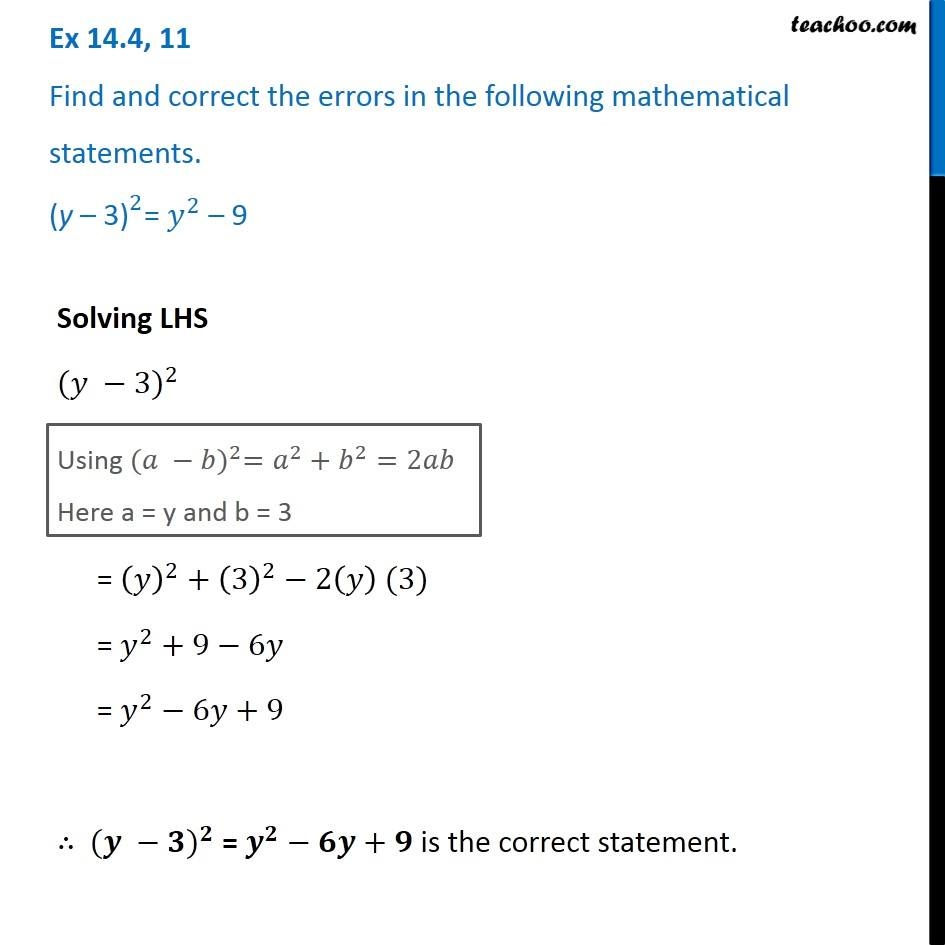Ex 14.4

Chapter 14 Class 8 Factorisation
Serial order wise### Transcript

Ex 14.4, 11 Find and correct the errors in the following mathematical statements. 〖"(y – 3)" 〗^2= 𝑦^2 – 9 Solving LHS 〖(𝑦 −3)〗^2 Using 〖(𝑎 −𝑏)〗^2=𝑎^2+𝑏^2=2𝑎𝑏 Here a = y and b = 3 Using 〖(𝑎 −𝑏)〗^2=𝑎^2+𝑏^2=2𝑎𝑏 Here a = y and b = 3